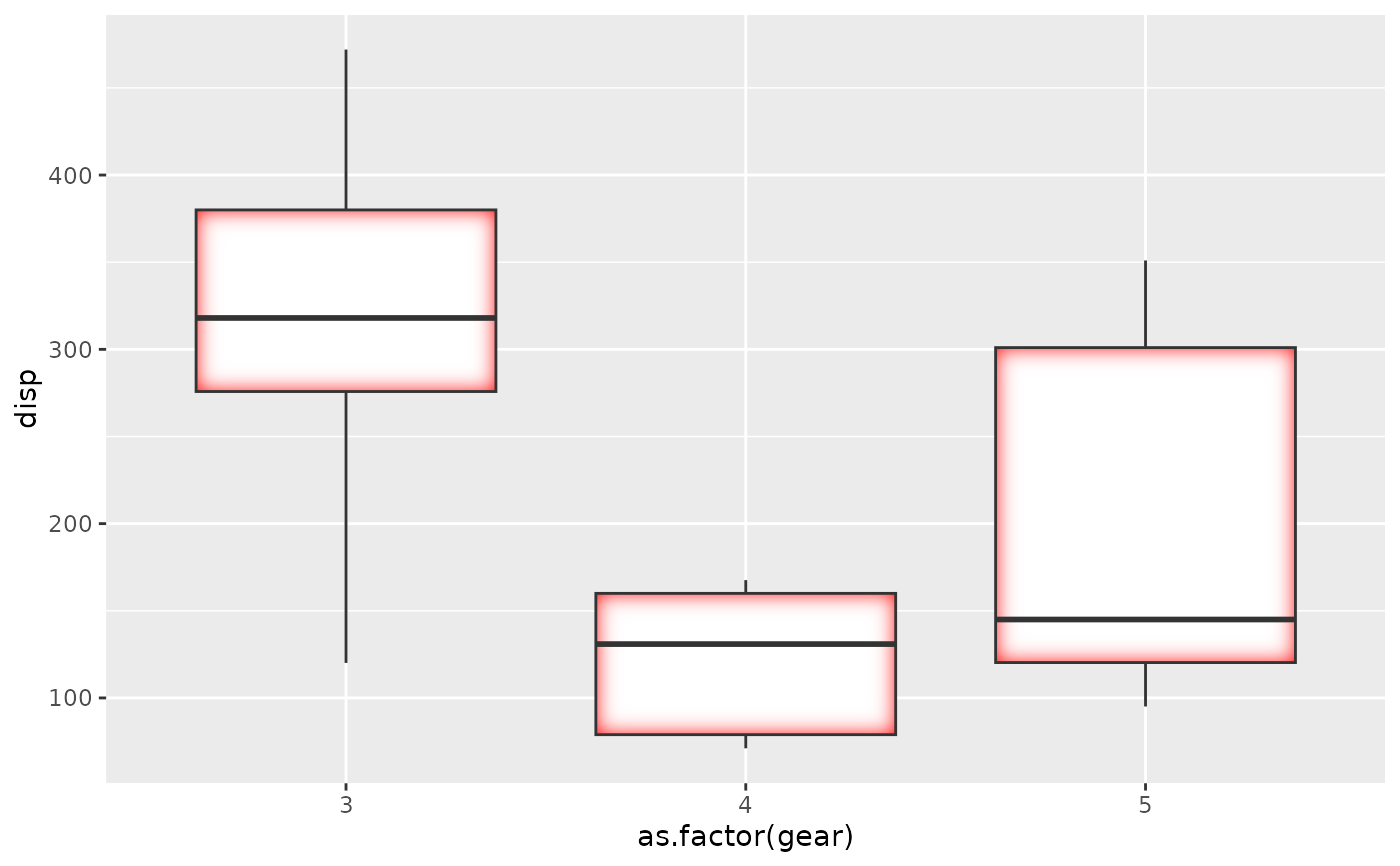This filter adds an inner glow to your layer with a specific colour and size. The best effect is often had by drawing the stroke separately so the glow is only applied to the fill.

with_inner_glow(x, colour = "black", sigma = 3, expand = 0, ...)

## Arguments

x

A ggplot2 layer object, a ggplot, a grob, or a character string naming a filter

colour

The colour of the glow

sigma

The standard deviation of the gaussian kernel. Increase it to apply more blurring. If a numeric it will be interpreted as given in pixels. If a unit object it will automatically be converted to pixels at rendering time

expand

...

Arguments to be passed on to methods. See the documentation of supported object for a description of object specific arguments.

## Value

Depending on the input, either a grob, Layer, list of Layers, guide, or element object. Assume the output can be used in the same context as the input.

Other glow filters: with_outer_glow()

## Examples

library(ggplot2)

ggplot(mtcars, aes(as.factor(gear), disp)) +
with_inner_glow(
geom_boxplot(),
colour = 'red',
sigma = 10
)# This gives a red tone to the lines as well which may not be desirable
# This can be fixed by drawing fill and stroke separately
ggplot(mtcars, aes(as.factor(gear), disp)) +
with_inner_glow(
geom_boxplot(colour = NA),
colour = 'red',
sigma = 10
) +
geom_boxplot(fill = NA)